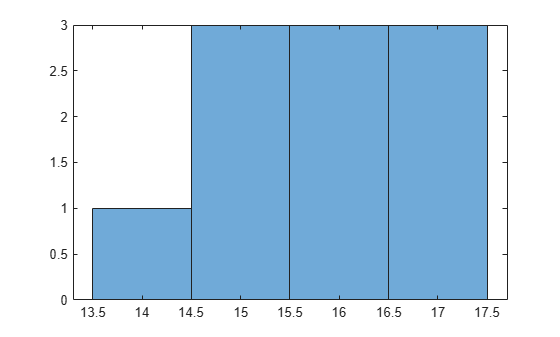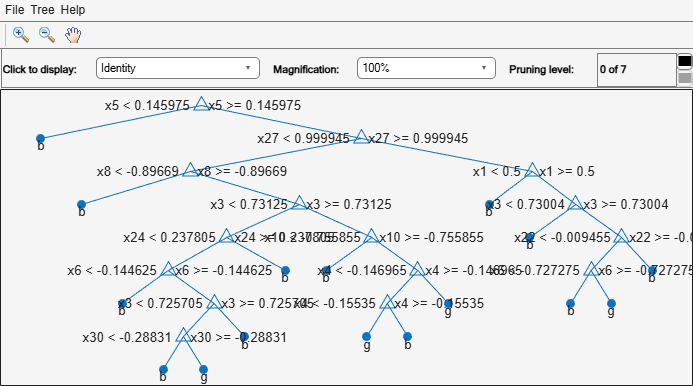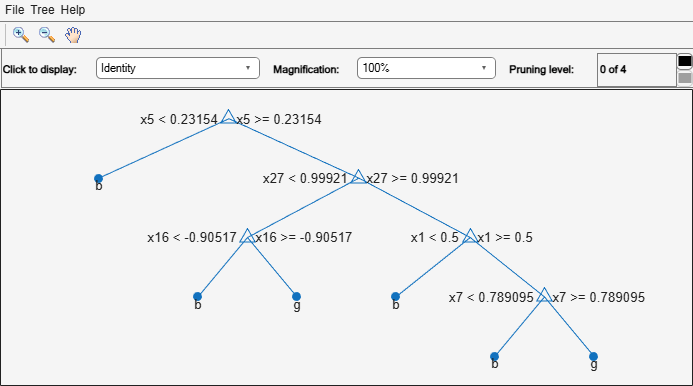Documentation

ClassificationTree class

Superclasses: CompactClassificationTree

Binary decision tree for classification

Description

A ClassificationTree object represents a decision tree with binary splits for classification. An object of this class can predict responses for new data using the predict method. The object contains the data used for training, so it can also compute resubstitution predictions.

Construction

Create a ClassificationTree object by using fitctree.

Properties

 BinEdges Bin edges for numeric predictors, specified as a cell array of p numeric vectors, where p is the number of predictors. Each vector includes the bin edges for a numeric predictor. The element in the cell array for a categorical predictor is empty because the software does not bin categorical predictors.The software bins numeric predictors only if you specify the 'NumBins' name-value pair argument as a positive integer scalar when training a model with tree learners. The BinEdges property is empty if the 'NumBins' value is empty (default).You can reproduce the binned predictor data Xbinned by using the BinEdges property of the trained model mdl.X = mdl.X; % Predictor data Xbinned = zeros(size(X)); edges = mdl.BinEdges; % Find indices of binned predictors. idxNumeric = find(~cellfun(@isempty,edges)); if iscolumn(idxNumeric) idxNumeric = idxNumeric'; end for j = idxNumeric x = X(:,j); % Convert x to array if x is a table. if istable(x) x = table2array(x); end % Group x into bins by using the discretize function. xbinned = discretize(x,[-inf; edges{j}; inf]); Xbinned(:,j) = xbinned; endXbinned contains the bin indices, ranging from 1 to the number of bins, for numeric predictors. Xbinned values are 0 for categorical predictors. If X contains NaNs, then the corresponding Xbinned values are NaNs. CategoricalPredictors Categorical predictor indices, specified as a vector of positive integers. CategoricalPredictors contains index values corresponding to the columns of the predictor data that contain categorical predictors. If none of the predictors are categorical, then this property is empty ([]). CategoricalSplit An n-by-2 cell array, where n is the number of categorical splits in tree. Each row in CategoricalSplits gives left and right values for a categorical split. For each branch node with categorical split j based on a categorical predictor variable z, the left child is chosen if z is in CategoricalSplits(j,1) and the right child is chosen if z is in CategoricalSplits(j,2). The splits are in the same order as nodes of the tree. Find the nodes for these splits by selecting 'categorical' cuts from top to bottom in the CutType property. Children An n-by-2 array containing the numbers of the child nodes for each node in tree, where n is the number of nodes. Leaf nodes have child node 0. ClassCount An n-by-k array of class counts for the nodes in tree, where n is the number of nodes and k is the number of classes. For any node number i, the class counts ClassCount(i,:) are counts of observations (from the data used in fitting the tree) from each class satisfying the conditions for node i. ClassNames List of the elements in Y with duplicates removed. ClassNames can be a categorical array, cell array of character vectors, character array, logical vector, or a numeric vector. ClassNames has the same data type as the data in the argument Y. (The software treats string arrays as cell arrays of character vectors.) ClassProbability An n-by-k array of class probabilities for the nodes in tree, where n is the number of nodes and k is the number of classes. For any node number i, the class probabilities ClassProbability(i,:) are the estimated probabilities for each class for a point satisfying the conditions for node i. Cost Square matrix, where Cost(i,j) is the cost of classifying a point into class j if its true class is i (the rows correspond to the true class and the columns correspond to the predicted class). The order of the rows and columns of Cost corresponds to the order of the classes in ClassNames. The number of rows and columns in Cost is the number of unique classes in the response. This property is read-only. CutCategories An n-by-2 cell array of the categories used at branches in tree, where n is the number of nodes. For each branch node i based on a categorical predictor variable X, the left child is chosen if X is among the categories listed in CutCategories{i,1}, and the right child is chosen if X is among those listed in CutCategories{i,2}. Both columns of CutCategories are empty for branch nodes based on continuous predictors and for leaf nodes. CutPoint contains the cut points for 'continuous' cuts, and CutCategories contains the set of categories. CutPoint An n-element vector of the values used as cut points in tree, where n is the number of nodes. For each branch node i based on a continuous predictor variable X, the left child is chosen if X=CutPoint(i). CutPoint is NaN for branch nodes based on categorical predictors and for leaf nodes. CutPoint contains the cut points for 'continuous' cuts, and CutCategories contains the set of categories. CutType An n-element cell array indicating the type of cut at each node in tree, where n is the number of nodes. For each node i, CutType{i} is: 'continuous' — If the cut is defined in the form X < v for a variable X and cut point v.'categorical' — If the cut is defined by whether a variable X takes a value in a set of categories.'' — If i is a leaf node. CutPoint contains the cut points for 'continuous' cuts, and CutCategories contains the set of categories. CutPredictor An n-element cell array of the names of the variables used for branching in each node in tree, where n is the number of nodes. These variables are sometimes known as cut variables. For leaf nodes, CutPredictor contains an empty character vector. CutPoint contains the cut points for 'continuous' cuts, and CutCategories contains the set of categories. CutPredictorIndex An n-element array of numeric indices for the variables used for branching in each node in tree, where n is the number of nodes. For more information, see CutPredictor. ExpandedPredictorNames Expanded predictor names, stored as a cell array of character vectors. If the model uses encoding for categorical variables, then ExpandedPredictorNames includes the names that describe the expanded variables. Otherwise, ExpandedPredictorNames is the same as PredictorNames. HyperparameterOptimizationResults Description of the cross-validation optimization of hyperparameters, stored as a BayesianOptimization object or a table of hyperparameters and associated values. Nonempty when the OptimizeHyperparameters name-value pair is nonempty at creation. Value depends on the setting of the HyperparameterOptimizationOptions name-value pair at creation: 'bayesopt' (default) — Object of class BayesianOptimization'gridsearch' or 'randomsearch' — Table of hyperparameters used, observed objective function values (cross-validation loss), and rank of observations from lowest (best) to highest (worst) IsBranchNode An n-element logical vector that is true for each branch node and false for each leaf node of tree. ModelParameters Parameters used in training tree. To display all parameter values, enter tree.ModelParameters. To access a particular parameter, use dot notation. NumObservations Number of observations in the training data, a numeric scalar. NumObservations can be less than the number of rows of input data X when there are missing values in X or response Y. NodeClass An n-element cell array with the names of the most probable classes in each node of tree, where n is the number of nodes in the tree. Every element of this array is a character vector equal to one of the class names in ClassNames. NodeError An n-element vector of the errors of the nodes in tree, where n is the number of nodes. NodeError(i) is the misclassification probability for node i. NodeProbability An n-element vector of the probabilities of the nodes in tree, where n is the number of nodes. The probability of a node is computed as the proportion of observations from the original data that satisfy the conditions for the node. This proportion is adjusted for any prior probabilities assigned to each class. NodeRisk An n-element vector of the risk of the nodes in the tree, where n is the number of nodes. The risk for each node is the measure of impurity (Gini index or deviance) for this node weighted by the node probability. If the tree is grown by twoing, the risk for each node is zero. NodeSize An n-element vector of the sizes of the nodes in tree, where n is the number of nodes. The size of a node is defined as the number of observations from the data used to create the tree that satisfy the conditions for the node. NumNodes The number of nodes in tree. Parent An n-element vector containing the number of the parent node for each node in tree, where n is the number of nodes. The parent of the root node is 0. PredictorNames Cell array of character vectors containing the predictor names, in the order which they appear in X. Prior Numeric vector of prior probabilities for each class. The order of the elements of Prior corresponds to the order of the classes in ClassNames. The number of elements of Prior is the number of unique classes in the response. This property is read-only. PruneAlpha Numeric vector with one element per pruning level. If the pruning level ranges from 0 to M, then PruneAlpha has M + 1 elements sorted in ascending order. PruneAlpha(1) is for pruning level 0 (no pruning), PruneAlpha(2) is for pruning level 1, and so on. PruneList An n-element numeric vector with the pruning levels in each node of tree, where n is the number of nodes. The pruning levels range from 0 (no pruning) to M, where M is the distance between the deepest leaf and the root node. ResponseName A character vector that specifies the name of the response variable (Y). RowsUsed An n-element logical vector indicating which rows of the original predictor data (X) were used in fitting. If the software uses all rows of X, then RowsUsed is an empty array ([]). ScoreTransform Function handle for transforming predicted classification scores, or character vector representing a built-in transformation function. none means no transformation, or @(x)x. To change the score transformation function to, for example, function, use dot notation. For available functions (see fitctree), enterMdl.ScoreTransform = 'function';You can set a function handle for an available function, or a function you define yourself by enteringtree.ScoreTransform = @function; SurrogateCutCategories An n-element cell array of the categories used for surrogate splits in tree, where n is the number of nodes in tree. For each node k, SurrogateCutCategories{k} is a cell array. The length of SurrogateCutCategories{k} is equal to the number of surrogate predictors found at this node. Every element of SurrogateCutCategories{k} is either an empty character vector for a continuous surrogate predictor, or is a two-element cell array with categories for a categorical surrogate predictor. The first element of this two-element cell array lists categories assigned to the left child by this surrogate split, and the second element of this two-element cell array lists categories assigned to the right child by this surrogate split. The order of the surrogate split variables at each node is matched to the order of variables in SurrogateCutPredictor. The optimal-split variable at this node does not appear. For nonbranch (leaf) nodes, SurrogateCutCategories contains an empty cell. SurrogateCutFlip An n-element cell array of the numeric cut assignments used for surrogate splits in tree, where n is the number of nodes in tree. For each node k, SurrogateCutFlip{k} is a numeric vector. The length of SurrogateCutFlip{k} is equal to the number of surrogate predictors found at this node. Every element of SurrogateCutFlip{k} is either zero for a categorical surrogate predictor, or a numeric cut assignment for a continuous surrogate predictor. The numeric cut assignment can be either –1 or +1. For every surrogate split with a numeric cut C based on a continuous predictor variable Z, the left child is chosen if Z

Methods

 compact Compact tree crossval Cross-validated decision tree cvloss Classification error by cross validation prune Produce sequence of classification subtrees by pruning resubEdge Classification edge by resubstitution resubLoss Classification error by resubstitution resubMargin Classification margins by resubstitution resubPredict Predict resubstitution labels of classification tree

Inherited Methods

 edge Classification edge loss Classification error margin Classification margins predict Predict labels using classification tree predictorImportance Estimates of predictor importance for classification tree surrogateAssociation Mean predictive measure of association for surrogate splits in classification tree view View classification tree

Copy Semantics

Value. To learn how value classes affect copy operations, see Copying Objects (MATLAB).

Examples

collapse all

Grow a classification tree using the ionosphere data set.

tc = fitctree(X,Y)
tc =
ClassificationTree
ResponseName: 'Y'
CategoricalPredictors: []
ClassNames: {'b'  'g'}
ScoreTransform: 'none'
NumObservations: 351

Properties, Methods

You can control the depth of the trees using the MaxNumSplits, MinLeafSize, or MinParentSize name-value pair parameters. fitctree grows deep decision trees by default. You can grow shallower trees to reduce model complexity or computation time.

Load the ionosphere data set.

The default values of the tree depth controllers for growing classification trees are:

• n - 1 for MaxNumSplits. n is the training sample size.

• 1 for MinLeafSize.

• 10 for MinParentSize.

These default values tend to grow deep trees for large training sample sizes.

Train a classification tree using the default values for tree depth control. Cross-validate the model by using 10-fold cross-validation.

rng(1); % For reproducibility
MdlDefault = fitctree(X,Y,'CrossVal','on');

Draw a histogram of the number of imposed splits on the trees. Also, view one of the trees.

numBranches = @(x)sum(x.IsBranch);
mdlDefaultNumSplits = cellfun(numBranches, MdlDefault.Trained);

figure;
histogram(mdlDefaultNumSplits)view(MdlDefault.Trained{1},'Mode','graph')The average number of splits is around 15.

Suppose that you want a classification tree that is not as complex (deep) as the ones trained using the default number of splits. Train another classification tree, but set the maximum number of splits at 7, which is about half the mean number of splits from the default classification tree. Cross-validate the model by using 10-fold cross-validation.

Mdl7 = fitctree(X,Y,'MaxNumSplits',7,'CrossVal','on');
view(Mdl7.Trained{1},'Mode','graph')Compare the cross-validation classification errors of the models.

classErrorDefault = kfoldLoss(MdlDefault)
classErrorDefault = 0.1140
classError7 = kfoldLoss(Mdl7)
classError7 = 0.1254

Mdl7 is much less complex and performs only slightly worse than MdlDefault.

expand all

References

 Breiman, L., J. Friedman, R. Olshen, and C. Stone. Classification and Regression Trees. Boca Raton, FL: CRC Press, 1984.